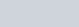•
•
•
•
•
•
•
•

# PERCENTAGE PROBLEMS  TRICKS

In today’s article, we are going to talk about some important arithmetic formulae on percentage, which may be very helpful to you, in all types of competitive examinations.# Some important sutras

1). If two values are respectively x% and y% more than the third value, then the first is the =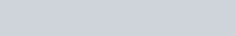2). If the two values are respectively x% and y% more than the third value, the second is the=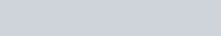3). If the two values are respectively x% and y% less than the third value, then the second is the=4). If the two values are respectively x% and y% less than the third value, then the first is the=5). If A is x% of C and B is y% of C then A is =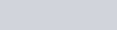6). If x% of quantity is taken by the first,y% of remaining is taken by the second and z% of remaining is taken by the third person, now if ₹A is left in the fund, then the fund was in the beginning=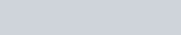7). If A is the initial amount in the fund and x% is taken by the first,y% is taken by the second and z% is taken by the third person then the amount left in the fund is =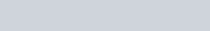8). If the initial amount is A, then x% of the initial amount is added to the initial amount, then y% of the increased amount is added to the amount, and then z% of the increased amount added then the initial amount become =9). If the original population of a town is P and the annual increase is r% then the population after n years will be=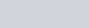10). If the present population of a town is P, and the annual increase is r% then the population was n years ago will be=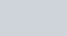11). If the original population of a town is P, and annual decrease is r% then population after n years will be=12). If the annual decrease in the population of a town is r%, and the present population is P, then the population of the town in n years ago will be=13). The population of a city is P, it increases/decreases by x% in the first year,y% in the second year, and z% in the third year. then the population after the three years will be=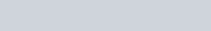14). If the population of a town is increase/decrease by x% in the first year,y% in the second year, and z% in the third year, and after the three years the population of the town is to be noted P then the population of the town in the beginning is =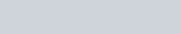15). If the population of the town is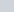, and in the town, male increased by x% and females increased by y% then the population become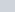, then the no. Of males and females in the town is given as below=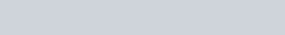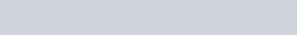16). If the value is decreased/decreased successively x% and y% then the net decrease is as follows=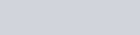17). If one number is decreased/increased by x% and the second number is decreased/increased by y% then the effect on the product is =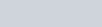18). The passing marks in an examination is x% and a student secures y marks and he fails by z marks, then the maximum marks of the examination are =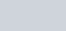19). A student scores x% marks, and fails by a mark, another student who has scored y% marks and he gets b marks more than the minimum required marks to be pass, then max marks of the examination is =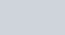20). In an examination x% fails in physics, y% failed in cosmology and z% students failed in both the subjects, then % of students who have passed both exams is =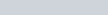21). A man spends x% of his income, his income is increased by y% and his expenditure also increased by z% then the % increased in his savings is =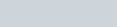22). If x% of objects is one type, the remaining y% is of the second type, and remaining z% is the third type, and the value of remaining objects is A then the total no of objects is =23). The producer of a goods makes a profit/loss of x%, the whole seller makes the profit of y%, and the retailer makes the profit of z% if the retailer sold it for Rs A, then the producing cost of the goods is =24). In L liters of x% sulphuric acid solution, the amount of water to be added/removed to make the y% of the acidic solution is =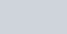Note: here x% is always greater than y% if H2O is added, if H2O is removed then y is greater.

25). A certain amount of solvent B is added to the solution of A and B of amount M to change the % amount of A to ∆A, then the amount of B to be added=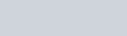26). If the original value of a trust is A and the new value is trust is B, then increase or decrease in the consumption such that the expenditure is unaffected is =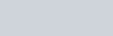27). Splitting of a number N into two parts such that one part is x% of other, then the split two parts is=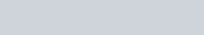28). If L liter of water is poured into a tank, but it is still x% empty then the amount of water should be added to fill it up to brim = L×x/(100-x) and capacity of tank is=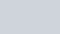29). If x%, y%, and z% is successively three discount are given then a net single discount is=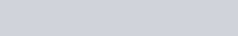30). If x% houses contain two or more people and those houses which contain only one male is y%, then % of all houses which contains exactly one female with no male is =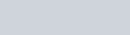31).  If the monthly income of A is x% more than that of B, monthly income of B is y% less than that of C, if the difference between the monthly income of A and C is M then the monthly income of B and C is =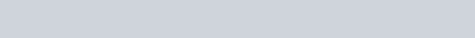32).  Mass of two boys A and B is in the ratio of a:b, if the mass of A is increased by x% then the total mass becomes M, if the mass of B is increased by y% then the total mass become=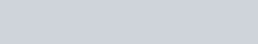33). If a person spends x% of his monthly income on the dance bar, and y% of remaining on worship, then after he saves Rs A, then the monthly income of the person is = A×100^2/(100-x)(100-y)
The monthly amount spent on the dance bar is =Ax×100/(100-x)(100-y)
Monthly amount spent on worship is=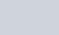34). If r% is decreased in the value of an object then a person buy A kg more objects in Rs x then the cost of the object is =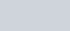35). In the election of two candidates, one gets x% votes of total votes but lose by y votes, then the total no of votes is =36). In an examination between A boys and B-girls,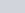Boyes pass andgirls get passed, then % of total passed students are =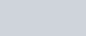37). If the value of any object is increased by x% and then decreased by x%, then resultant is always get decreased then resultant is given as=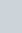38). Convert x% in fraction =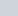39).  Convert x% in decimal= 0.0x

40).  Convertin percentage =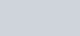41). If x% of A is equal to the y% of B, then z% of A is =